A Study on Square Waves   Just what is a square wave anyhow?!? A square wave is a waveform that is built up from a series of harmonics derived from the fundamental frequency. A true square wave will have 3rd, 5th, 7th, 9th, 11th, 13th and 15th harmonics. The rise and fall is very abrupt, straight up and straight down. For an audio signal, all of these combined odd order harmonics would not be considered to be a pleasant sound by most. Here is a comparison of a sine wave with a square wave: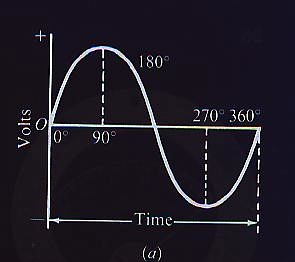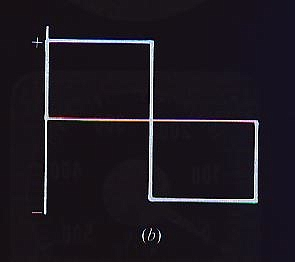The sine wave has a smooth rise and fall, and rounded peaks. The square wave is exactly what its name implies - square. So, how does a square wave get built up from a sine wave? By adding a series of harmonics until a square wave is achieved.   Here is what you get when a third harmonic is added to the fundamental: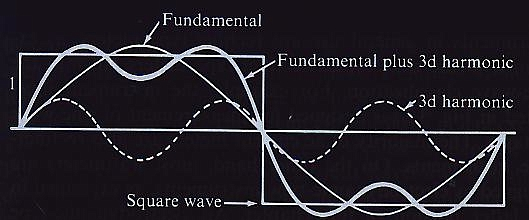Here is what you get when you add a fifth harmonic to that: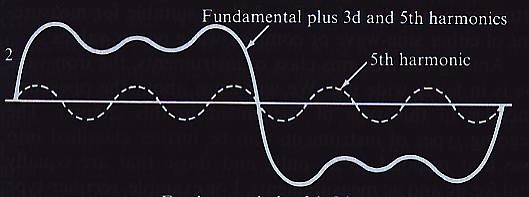Here is what you get when you add a seventh harmonic to that: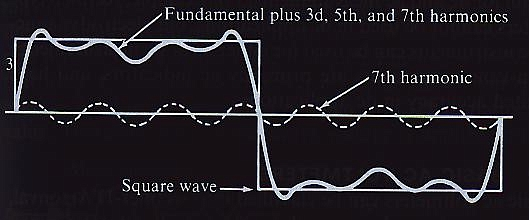The strength of each progressively higher harmonic decreases, as can be seen from the figure below.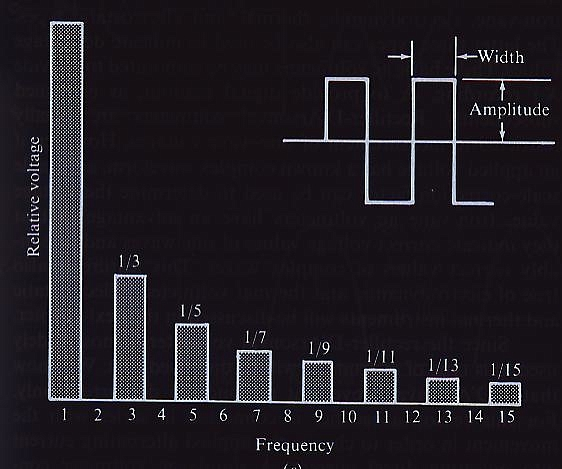So as you can see, as you add more and more odd harmonics, the wave form gets more and more square until you eventually arrive at a true square wave. So, we find that square waves are packed with harmonics, but not the right ones for general audio use.